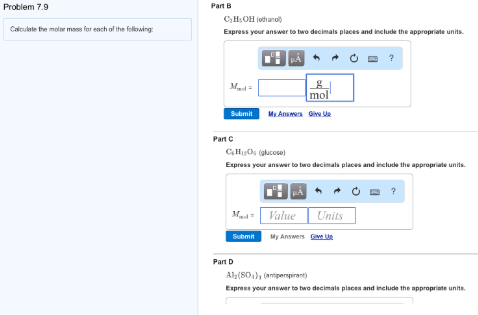# Problem: Calculate the molar mass for each of the following: Part BC2H5OH (ethanol)Express your answer to two decimals places and include the appropriate units. Part C C6H12O6 (glucose) Express your answer to two decimals places and include the appropriate units. Part D Al2(SO4)3 (antiperspirant) Express your answer to two decimals places and include the appropriate units

###### FREE Expert Solution###### Problem Details

Calculate the molar mass for each of the following:

Part B

C2H5OH (ethanol)

Express your answer to two decimals places and include the appropriate units.

Part C

C6H12O6 (glucose)

Express your answer to two decimals places and include the appropriate units.

Part D

Al2(SO4)3 (antiperspirant)

Express your answer to two decimals places and include the appropriate units